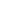# Introduction to Quantum Physics

Quantum physics underpins all of modern science. We could not understand chemistry, biology, nuclear phenomena, computer chips and solar energy if we could not rely on quantum theory. In this short course, we shall explain the reasons for the birth of quantum physics, and describe some of the basic principles and concepts that form the foundations of this field of physics. And we shall do this without using complex mathematics.

• Face-to-Face

#### COURSE OUTLINE

• A brief introduction to ‘classical’ physics, the physics before the 20th century. It worked just fine, why did we need quantum physics?
• Light: what is it made of: particles or waves? Discussion of wave interference and diffraction phenomena and experiments, including the all-important double slit experiment.
• Experiments during the first part of the 20th century that lead to the development of quantum physics, such as the problem of black-body radiation, the photoelectric effect, the Compton experiment. Introduction to photons.
• The measurement of optical spectra, absorption and emission of light. The unexpected hydrogen spectrum. How is light generated?
• Discovery of the electron; the Rutherford model of the atom; the Bohr atom. How do atoms emit light? Introduction to energy levels and quantum numbers.
• De Broglie and the matter waves; the double-slit experiment again, but this time ‘a surprise’; wave-like behaviour of electrons; electron diffraction. Heisenberg’s uncertainty principle.
• Schrodinger’s wave equation. Waves of probability; spin; Pauli’s exclusion principle; quantum entanglement, the EPR ‘paradox’, Schrodinger’s cat
• What does all this mean? Various interpretations of quantum physics.

#### LEARNING OUTCOMES

By the end of this course, students should be able to:

1. Discuss some of the important phenomena that lead to the development of quantum physics.
2. Discuss interference and diffraction of waves, and the notion of the wave-particle nature of light.
3. Discuss the Schrodinger’s time independent wave equation and its significance.
4. Discuss various interpretations of quantum physics.
\$171 Limited / \$154

<p>Quantum physics underpins all of modern science. We could not understand chemistry, biology, nuclear phenomena, computer chips and solar energy if we could not rely on quantum theory. In this

...
03 Feb
Mo Tu We Th Fr Sa SuInterested in this course but can't attend? Please join the waiting list by clicking .

### Course testimonials:

• Excellent presentation with good slides and handouts for each session. I enjoyed it very much.